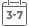# Sound Propagation: An Impedance Based Approach

• Publish Date: 2010-10-04
• Binding: Hardcover
• Author: Yang-Hann Kim
• Sale
• \$135.70
• Regular price \$148.97In Sound Propagation: An Impedance Based Approach, Professor Yang-Hann Kim introduces acoustics and sound fields by using the concept of impedance. Kim starts with vibrations and waves, demonstrating how vibration can be envisaged as a kind of wave, mathematically and physically. One-dimensional waves are used to convey the fundamental concepts. Readers can then understand wave propagation in terms of characteristic and driving point impedance. The essential measures for acoustic waves, such as dB scale, octave scale, acoustic pressure, energy, and intensity, are explained. These measures are all realized by one-dimensional examples, which provide mathematically simplest but clear enough physical insights.

Kim then moves on to explaining waves on a flat surface of discontinuity, demonstrating how propagation characteristics of waves change in space when there is a distributed impedance mismatch. Next is a chapter on radiation, scattering, and diffraction, where Kim shows how these topics can be explained in a unified way, by seeing the changes of waves due to spatially distributed impedance. Lastly, Kim covers sound in closed space, which is considered to be a space that is surrounded by spatially distributed impedance, and introduces two spaces: acoustically large and small space. The bulk of the book is concerned with introducing core fundamental concepts, but the appendices are included as the essentials as well to cover other important topics to extend learning.

• Offers a less mathematically-intensive means to understand the subject matter
• Provides an excellent launching point for more advanced study or for review of the basics
• Based on classroom tested materials developed over the course of two decades